Bihar Board 12th Physics Objective Questions and Answers

## Bihar Board 12th Physics Objective Answers Chapter 14 Semiconductor Electronics: Materials, Devices and Simple CircuitsQuestion 1.
At absolute zero, Si acts as a
(a) metal
(b) Semiconductor
(c) insulator
(d) None of these
(c) insulator

Question 2.
In good conductors of electricity the type of bonding that exist is
(a) van der Walls
(b) covalent
(c) ionic
(d) metallic
(d) metallic

Question 3.
The manifestation of band structure in solids is due to
(a) Heisenberg uncertainty principle
(b) Pauli’s exclusion principle
(c) Bohr’s correspondence principle
(d) Boltzmann law
(b) Pauli’s exclusion principleQuestion 4.
The probability of electrons to be found in the conduction band of an intrinsic semicondutor of finite temperature
(a) increases exponentially with increasing band gap
(b) decreases exponentially with increasing band gap
(c) decreases with increasing temperature
(d) is independent of the temperature and band gap
(b) decreases exponentially with increasing band gap

Question 5.
In n-type semiconductor when all donor states are filled, then the net charge density in the donor states becomes
(a) 1
(b) > 1
(c) < 1, but not zero
(d) zero
(b) > 1

Question 6.
A semiconductor has equal electron and hole concentration of 6 x 108 per m3. On doping with certain impurity, electron concentration increases to 9 x 1012 per m3. The new hole concentration is
(a) 2 x 104 per m3
(b) 2 x 102 per m3
(c) 4 x 104 per m3
(d) 4x 102 perm3
(c) 4 x 104 per m3Question 7.
The dominant mechanism for motion of charge carriers in forward and reverse biased silicon p-n junction are
(a) drift in forward bias, diffusion in reverse bias
(b) diffusion in forward bias, drifit in reverse bias
(c) diffusion in both forward and reverse bias
(d) drift in both forward and reverse bias
(b) diffusion in forward bias, drifit in reverse bias

Question 8.
Region without free electrons and holes in a p-n junction is
(a) n-region
(b) p-region
(c) depletion region
(d) none of these
(c) depletion region

Question 9.
Potential barrier developed in a junction diode opposes the flow of
(a) minority carrier in both regions only
(b) majority carriers only
(c) electrons in p region
(d) holes in p region
(b) majority carriers onlyQuestion 10.
A potential barrier of 0.3 V exists across a p-n junction. If the depletion region is 1 pm wide, what is the intensity of electric field in this region ?
(a) 2 x 105 V m-1
(b) 3 x 105 V m-1
(c) 4 x 105 V m-1
(d) 5 x 105 V m-1
(b) 3 x 105 V m-1

Question 11.
A forward-biased diode is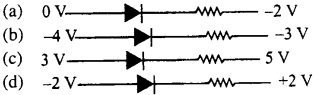(a)

Question 12.
When the voltage drop across a p-n junction diode is increased from 0.65 V to 0.70 V, the change in the diode current is 5 mA. The dynamic resistance of the diode is
(a) 5 Ω
(b) 10 Ω
(c) 20 Ω
(d) 25 Ω
(b) 10 ΩQuestion 13.
In the circuit shown if current for the diode is 20 pA, the potential difference across the diode is(a) 2 V
(b) 4.5 V
(c) 4 V
(d) 2.5 V
(c) 4 V

Question 14.
The equivalent resistance of the circuit shown in figure between the points A and B if VA < VB is(a) 10 Ω
(b) 20 Ω
(c) 5 Ω
(d) 40 Ω
(b) 20 Ω

Question 15.
In the question number 34, the equivalent resistance between the points A and B, if VA > VB is
(a) 10 Ω
(b) 20 Ω
(c) 30 Ω
(d) 15 Ω
(a) 10 ΩQuestion 16.
In a half wave rectifier circuit operating from 50 Hz mains frequency, the fundamental frequency in the ripple would be
(a) 25 Hz
(b) 50 Hz
(c) 70.7 Hz
(d) 100 Hz
(b) 50 Hz

Question 17.
What happens during regulation action of a Zener diode?
(a) The current through the series resistance (RS) changes
(b) The resistance offered by the Zener changes
(c) The Zener resistance is constant
(d) Both (a) and (b)
(d) Both (a) and (b)

Question 18.
From the Zener diode circuit shown in figure, the current through the Zener diode is(a) 34 mA
(b) 31.5 mA
(c) 36.5 mA
(d) 2.5 mA
(b) 31.5 mAQuestion 19.
A p-n photodiode is fabricated from a semiconductor with a band gap of 2.5 eV. The signal wavelength is
(a) 6000 A
(b) 6000 nm
(c) 4000 nm
(d) 5000 A
(d) 5000 A

Question 20.
The transfer characteristics of a base biased transistor has the operation regions, namely, cutoff, active region and saturation region. For using the transistor as an amplifier it has to operate in the
(a) active region
(b) cutoff region
(c) saturation region
(d) cutoff and saturation
(a) active region

Question 21.
The emitter of transistor is doped the heaviest because it
(a) acts as a supplier of charge carriers
(b) dissipates maximum power
(c) has a larger resistance
(d) has a small resistance
(a) acts as a supplier of charge carriersQuestion 22.
The heavily and lightly doped regions of a bipolar junction transistor are respectively
(a) base and emitter
(b) base and collector
(c) emitter and base
(d) collector and emitter
(c) emitter and base

Question 23.
An oscillator is nothing but an amplifier with
(a) larger gain
(b) positive feedback
(c) no feedback
(d) negative feedback
(b) positive feedback

Question 24.
The current amplification factor a of a common base transistor and the current amplification factor 3 of a common emitter transistor are not related by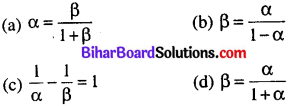(d)

Question 25.
If a and 3 are the current gain in the CB and CE configurations respectively of the transistor circuit, then $$\frac{\beta-\alpha}{\alpha \beta}=$$
(a) zero
(b) 1
(c) 2
(d) 0.5
(b) 1Question 26.
A transistor connected in common emitter mode, the voltage drop across the collector is 2 V and 3 is 50, the base current if Rc is 2 kΩ is
(a) 40 μA
(b) 20 μA
(c) 30 μA
(d) 15 μA
(b) 20 μA

Question 27.
The current gain for a common emitter amplifier is 69. If the emitter current is 7 mA, the base current is
(a) 0.1 m A
(b) 1 mA
(c) 0.2 m A
(d) 2 mA
(a) 0.1 m A

Question 28.
The ac current gain of a transistor is 120. What is the change in the collector current in the transistor whose base current changes by 100 μA ?
(a) 6 mA
(b) 12 mA
(c) 3 mA
(d) 24 mA
(b) 12 mAQuestion 29.
In an n-p-n circuit transistor, the collector current is 10 mA. If 80% electron emitted reach the collector, then
(a) the emitter current will be 7.5 mA
(b) the emitter current will be 12.5 mA
(c) the base current will be 3.5 mA
(d) the base current will be 1.5 mA
(b) the emitter current will be 12.5 mA

Question 30.
A transistor has a current gain of 30. If the collector resistance is 6 kΩ, input resistance is 1 kΩ, it voltage gain is
(a) 90
(b) 180
(c) 45
(d) 360
(b) 180

Question 31.
In a transistor connected in common emitter mode, Rc =4 kΩ, R1 = 1 kΩ, Ic = 1 mA and IB = 20μA. The voltage gain is
(a) 100
(b) 200
(c) 300
(d) 400
(b) 200Question 32.
In an n-p-n transistor 1010 electron enter the emitter in 10-6 s. If 2% of the electrons are lost in the base, the current amplification factor is
(a) 0.02
(b) 7
(c) 33
(d) 4.9
(a) 0.02

Question 33.
If a change of 100 pA in the base current of an n-p-n transistor causes a change of 10 mA in its collector current, its ac current gain is
(a) 50
(b) 100
(c) 200
(d) 150
(b) 100

Question 34.
What is the voltage gain in a common emitter amplifier, where input resistance is 3Ω and load resistance 24 Ω and β = 61 ?
(a) 8.4
(b) 488
(c) 240
(d) 0
(b) 488Question 35.
An amplifier has a voltage gain of 100. The voltage gain in dB is
(a) 20 dB
(b) 40 dB
(c) 30dB
(d) 50dB
(b) 40 dB

Question 36.
Boolean algebra is essentially based on
(a) number
(b) truth
(c) logic
(d) symbol
(c) logic

Question 37.
The symbolic representation of four logic gates are given below. The logic symbols for OR, NOT and NAND gates are respectively.(a) (i v), (i), (ii i)
(b) (i v), (ii), (i)
(c) (i), (iii), (iv)
(d) (iii), (iv), (ii).
(b) (i v), (ii), (i)Question 38.
Study the circuit shown in the figure. Name the gate that the given circuit resembles.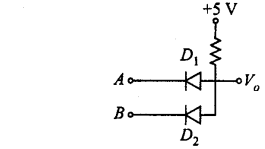(a) NAND
(b) AND
(c) OR
(d) NOR
(b) AND

Question 39.
In Boolean algebra if A = 1 and B = 0, then the value of A + $$\bar{B}$$ is
(a) A
(b) A.B
(c) A + B
(d) both (a) and (c)
(d) both (a) and (c)

Question 40.
What will be input of A and B for the Boolean expression $$(\overline{A+B}) \cdot(\overline{A \cdot B})=1 ?$$?
(a) (0, 0)
(b) (0, 1)
(c) (1, 0)
(d) (1, 1)
(a) (0, 0)Question 41.
The circuit given in figure, is equivalent to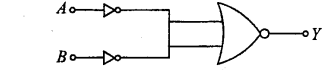(a) AND gate
(b) OR gate
(c) NOT gate
(d) NAND gate
(a) AND gate

Question 42.
The given truth table is for which logic gate ?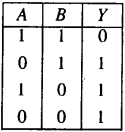(a) NAND
(b) XOR
(c) NOR
(d) OR
(a) NAND

Question 43.
The decimal equivalent of the binary number (11010.101) 2is
(a) 9.625
(b) 25.265
(c) 26.625
(d) 26.265
(d) 26.265Question 44.
Hole is
(a) an anti-particle of electron.
(b) a vacancy created when an electron leaves a covalent bond.
(c) absence of free electrons.
(d) an artificially created particle.
(b) a vacancy created when an electron leaves a covalent bond.

Question 45.
In the circuit shown in figure, if the diode forward voltage drop is 0.3 V, the voltage difference between A and B is
(a) 1.3 V
(b) 2.3 V
(c) 0
(d) 0.5V
(b) 2.3 V

Hints & Explanations

Question 6.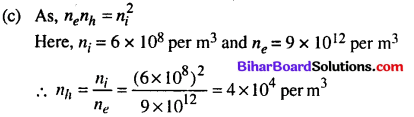Question 12.
(b) Dynamic resistance is $$r_{d}=\frac{\Delta V}{\Delta I}$$
Here, ΔV = 0.7 – 0.65 V = 0.05 V,
ΔI = 5 mA = 5 x 10-3 A
∴ $$r_{d}=\frac{0.05}{5 \times 10^{-3}}=10 \Omega$$Question 13.
(c) Since the diode is reversed biased, only drift current exists in circuit which is 20 pA.
Potential drop across 15 Ω resistor
= 15 Ω x 20 μA
= 300 μV = 0.0003 V
Potential difference across the diode
= 4 – 0.0003
= 3.99 = 4V

Question 14.
(b) When VA < VB, the diode gets reverse biased and offers infinite resistance. No current flows through the upper branch
∴ R = 20 Ω

Question 18.
(b) Here, RL = 5 x 103 Ω, V, = 220 V,
Zener voltage, VZ = 50 V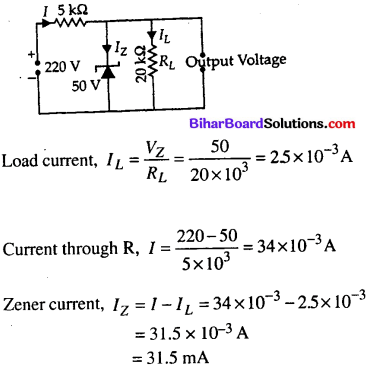Question 19.
(d) The detection occurs only when the energy of incident photon greater than or equal to the energy band gapQuestion 25.
(b) Since P = $$\beta=\frac{\alpha}{1-\alpha}$$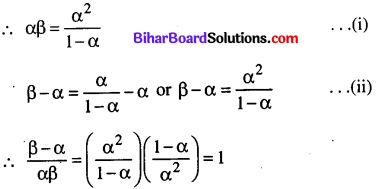Question 26.Question 27.
(a) Current gain, $$\beta=\frac{I_{C}}{I_{B}}$$
For common emitter configuration, IE = Ic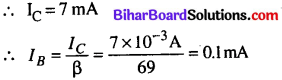Question 28.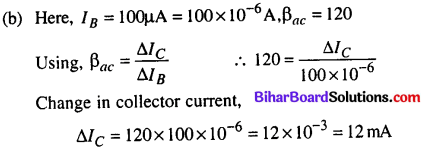Question 29.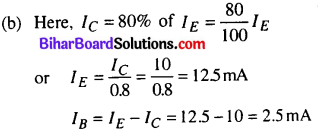Question 30.
(b) Voltage gain = current gain x resistance gain
= current gain $$\times \frac{R_{C}}{R_{I}}=30 \times \frac{6}{1}=180$$Question 31.
(b) Voltage gain,(a) As, $$I_{E}=\frac{n_{E} \times e}{t}$$(b) Here, $$\Delta I_{B}=100 \mu \mathrm{A}=100 \times 10^{-6} \mathrm{A}$$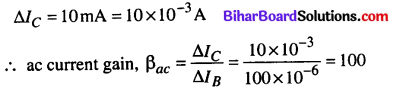(b) Voltage gain, $$A_{V}=\beta \frac{R_{o}}{R_{i}}=\frac{61 \times 24}{3}=488$$